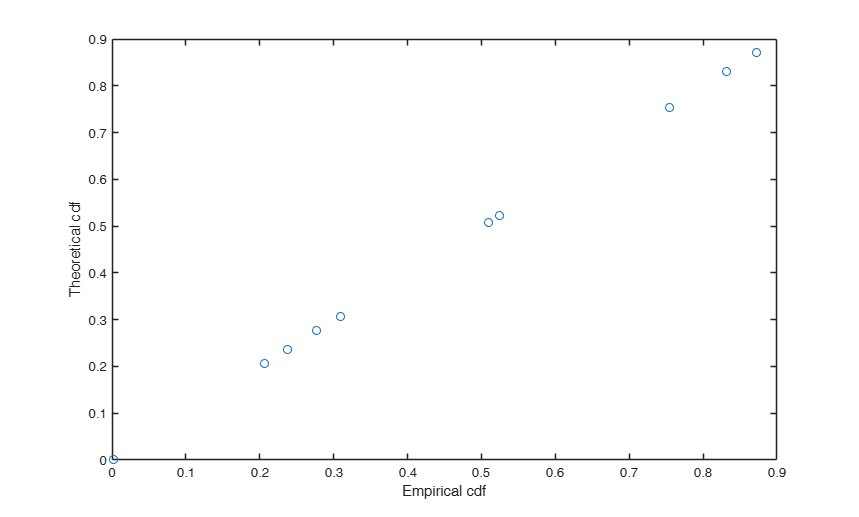# exactcdf

exactcdf finds exact p-values

## Syntax

• p=exactcdf(x)example
• p=exactcdf(x,empdist)example

## Description

Function for finding the exact cdf of each element in the vector x with respect to the empirical distribution, represented by the vector empdist, i.e. the generic element i of the output vector p is the result of:

$\frac{ \displaystyle \sum_{j=1}^K I_{empdist(j) \leq x_i}}{K}$ where $I$ is the indicator function and $K$ is the length of vector $empdist$

 p =exactcdf(x) exactcdf with just one input argument.

 p =exactcdf(x, empdist) exactcdf with two input arguments.

## Examples

expand all

### exactcdf with just one input argument.

k=1000;
x=randn(k,1);
p=exactcdf(x);

###exactcdf with two input arguments.

k=10;
x=randn(k,1);
K=100000;
empdist=randn(K,1);
% Compute empirical cdf for each element of vector x.
p=exactcdf(x,empdist);
% Compute theoretical cdf based on normcdf
pTheo=normcdf(x);
% Compare empirical cdf with theoretical cdf
plot(p,pTheo,'o')
xlabel('Empirical cdf')
ylabel('Theoretical cdf')## Related Examples

expand all

### Using exactcdf for calculating exact p-values.

k=10;
x=randn(k,1);
K=100000;
empdist=randn(K,1);
% Compute empirical cdf for each of element of vector x.
p=exactcdf(x,empdist);
% Compute exact p-values for an unilateral right-tailed test
pval_rt=1-p;
% Compute exact p-values for an unilateral left-tailed test
pval_lt=p;

## Input Arguments

### x — empirical replicates of a test. Vector.

Vetor of length k containing the empirical realization of a generic test

Data Types: double

### empdist — empirical distribution of the same test. Vector.

Vector of length $K$ generally with $K \geq k$ containig the empirical simulated distribution of the test. If this optional argument is not supplied the empirical distribution is taken from input vector x.

Example: randn(K,1) 

Data Types: double

## Output Arguments

### p —empirical cdf.  Vector

Vector with the same length of input vector x containing the empirical cdf of each element of input vector x. More precisely: $p(i)$ is computed as $\frac{ \displaystyle \sum_{j=1}^K I_{empdist(j) \leq x_i}}{K}$

Athey, S., Eckles, D., & Imbens, G. W. (2018). Exact p-values for network interference, "Journal of the American Statistical Association", Vol. 113, pp. 230-240.